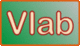online_muskingum_cunge_convergence_ratios:    Convergence ratios of the Muskingum-Cunge method This set of two online calculators: (1) Online_Muskingum_Cunge_convergence_ratios, and (2) Online_Muskingum_Cunge_convergence_ratios_practical, are intended to help the user of the Muskingum-Cunge method of flood routing choose the numerical parameters (grid resolution) that will provide sufficient accuracy for a given problem. The calculator Online_Muskingum_Cunge_convergence_ratios is recommended for use when a first approximation on convergence is indicated. This calculator inputs spatial resolution L/Δx, Courant number C, and weighting factor X. The calculator outputs amplitude and phase convergence ratios R1 and R2, respectively. The latter values should be as close to 1, but less than 1, as possible, e.g., 0.9995. The calculator Online_Muskingum_Cunge_convergence_ratios_practical is recommended for use when a second approximation on convergence is indicated. This calculator inputs hydraulic variables (mean velocity u, mean flow depth d, mean channel slope So, rating exponent β, and flood wave time-of-rise tr), and numerical parameters (reach length Δx and time interval Δt). The calculator outputs spatial resolution L/Δx, Courant number C, cell Reynolds number D, weighting factor X, amplitude convergence ratio R1, and phase convergence ratio R2. The convergence ratios should be as close to 1, but less than 1, as possible, e.g., 0.9995. Allowable range of values for Online_Muskingum_Cunge_convergence_ratios: Weighting factor:  0 ≤ X ≤ 1 Spatial resolution:  10 ≤ L /Δx ≤ 400 Courant number:  0.1 ≤ C ≤ 10 Allowable range of values for Online_Muskingum_Cunge_convergence_ratios_practical: Mean velocity:  0.1 ≤ u ≤ 10 [m/s] Mean flow depth:  0.1 ≤ d ≤ 100 [m] Mean channel slope:  0.00001 ≤ u ≤ 0.1 [m/m] Rating exponent:  1 ≤ β ≤ 5/3  •  [May leave blank to specify default β = 5/3] Flood wave time-to-rise:   1 ≤ tr ≤ 720 [hr] Reach length:   1 ≤ Δx ≤ 10000 [m] Time interval:   0.1 ≤ Δt ≤ 24 [hr] Thank you for running online_muskingum_cunge_convergence_ratios